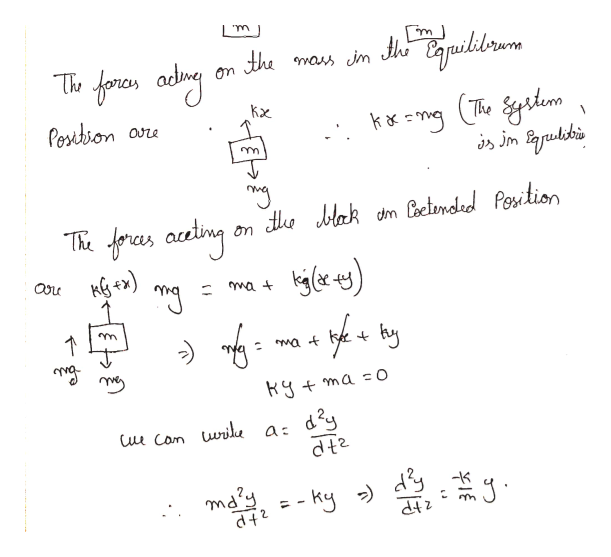# Find the equation of simple harmonic motion for a spring mass system where the mass is hanging off the ceiling with the help of a spring if the initial displacement is 1 ft above equilibrium with initial upward velocity of 2 ft/s. Assume w=1. Write in form y=Asin(x+b). Solve for A, x, and b.

Question
7 views

Find the equation of simple harmonic motion for a spring mass system where the mass is hanging off the ceiling with the help of a spring if the initial displacement is 1 ft above equilibrium with initial upward velocity of 2 ft/s. Assume w=1. Write in form y=Asin(x+b). Solve for A, x, and b.

check_circle

Step 1

Given information:

Step 2

Finding the simple harmonic equation:help_outlineImage Transcriptionclose'm mass im h uilibrwrs the The foros ding Posbion The isjm Brudekis Ym HJlak dm actndad Position fras acding on de m 1 d2y i Con wrike a: dt2 may-ky fullscreen
Step 3

Solving the different...

### Want to see the full answer?

See Solution

#### Want to see this answer and more?

Solutions are written by subject experts who are available 24/7. Questions are typically answered within 1 hour.*

See Solution
*Response times may vary by subject and question.
Tagged in

### Physics